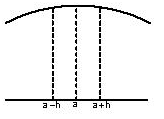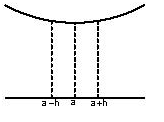Click to Chat

1800-1023-196

+91-120-4616500

CART 0

• 0

MY CART (5)

Use Coupon: CART20 and get 20% off on all online Study Material

ITEM
DETAILS
MRP
DISCOUNT
FINAL PRICE
Total Price: Rs.

There are no items in this cart.
Continue Shopping• Complete JEE Main/Advanced Course and Test Series
• OFFERED PRICE: Rs. 15,900
• View Details

```Revision Notes on Maxima and MinimaA function f(x) is said to have a local maximum at x = a if the value of f(a) is greater than all the values of f(x) in a small neighbourhood of x = a. Mathematically, f (a) > f (a – h) and f (a) > f (a + h) where h > 0, then a is called the point of local maximum.A function f(x) is said to have a local minimum at x = a, if the value of the function at x = a is less than the value of the function at the neighboring points of x = a. Mathematically, f (a) < f (a – h) and f (a) < f (a + h) where h > 0, then a is called the point of local minimum.

A point of local maximum or a local minimum is also called a point of local extremum.

A point where the graph of function is continuous and has a tangent line and where the concavity changes is called point of inflexion.

At the point of inflexion, either y” = 0 and changes sign or y” fails to exist.

At the point of inflexion, the curve crosses its tangent at that point.

A function cannot have point of inflexion and extrema at the same point.

Working rules to find points of local maxima and local minima:

1. First Derivative Test: If f'(a)  = 0 and f'(x)  changes its sign while passing through the point x = a, then

f(x) would have a local maximum at x = a if f'(a – 0)  > 0 and  f'(a + 0) < 0. It means that f'(x) should change its sign from positive to negative.

f(x) would have local minimum at x = a if f'(a – 0) < 0  and f'(a + 0) > 0 . It means that f'(x) should change its sign from negative to positive.

If f(x) doesn’t change its sign while passing through x = a, then f (x) would have neither a maximum nor minimum at x = a. e.g. f (x) = x3 doesn’t have any local maxima or minima at x = 0.

2. Second Derivative Test:

Let f(x) be a differentiable function on a given interval and let f'' be continuous at stationary point. Find f'(x) and solve the equation f'(x) = 0 given let x = a, b, … be solutions.

There can be two cases:

Case (i): If f''(a) <0 then f(a) is maximum.

Case (ii): If f ''(a) > 0 then f(a) is minimum.

In case, f''(a) = 0 the second derivatives test fails and then one has to go back and apply the first derivative test.

If f''(a) = 0 and a is neither a point of local maximum nor local minimum then a is a point of inflection.

3. nth Derivative Test for Maxima and Minima: Also termed as the generalization of the second derivative test, it states that if the n derivatives i.e. f '(a) = f''(a) = f'''(a) =………. = f n(a) = 0 and fn+1(a) ≠ 0 (all derivatives of the function up to order ‘n’ vanish and (n + 1)th order derivative does not vanish at x = a), then f (x) would have a local maximum or minimum at x = a iff n is odd natural number and that x = a would be a point of local maxima if fn+1 (a) < 0 and would be a point of local minima if fn+1 (a) > 0.

In some questions involving determination of maxima and minima, it might become difficult to decide whether f(x) actually changes its sign while passing through x = a and here, nth derivative test can be applied.

Global Minima & Maxima of f(x) in [a, b] is the least or the greatest value of the function f(x) in interval [a, b].

1. The function f(x) has a global maximum at the point ‘a’ in the interval I if f (a) ≥ f(x), for all x ∈ I.

2. Function f(x) has a global minimum at the point ‘a’ if f (a) ≤ f (x), for all x ∈ I.

Global Maxima Minima always occur either at the critical points of f(x) within [a, b] or at the end points of the interval.

Computation of Global Maxima and minima in maxima minima problems:

1. Compute the critical points of f(x) in (a, b). Let the various critical points be C1, C2, …. , Cn.

2. Next, compute the value of the function at these critical points along with the end points of the domain. Let us denote these values by f(C1), f(C2)………..f(Cn).

3. Now, compute M* = max{f(a), f(C1), f(C2)………..f(Cn), f(b)} and M** = min{f(a), f(C1), f(C2)………..f(Cn), f(b)}.Now M* is the maximum value of f(x) in [a, b] and M** is the minimum value of f(x) in [a, b].

In order to find global maxima or minima in open interval (a, b) proceed as told above and after the first two steps, compute

M1 = max{f(C1), f(C2)………..f(Cn)} and

M2 = min{f(C1),f(C2)………..f(Cn)}.

Now if x approaches a- or if x approaches b- , the limit of f(x) > M1 or its limit f(x) < M1 would not have global maximum (or global minimum) in (a, b) but if as x approaches a- and x approaches b- , lim f(x) < M1 and lim f(x) > M2, then M1 and M2 will respectively be the global maximum and global minimum of f(x) in (a,b).

If f(x) is a continuous function on a closed bounded interval [a,b], then f(x) will have a global maximum and a global minimum on [a,b]. On the other hand, if the interval is not bounded or closed, then there is no guarantee that a continuous function f(x) will have global extrema.

If f(x) is differentiable on the interval I, then every global extremum is a local extremum or an end point extremum.

```### Course Features

• 728 Video Lectures
• Revision Notes
• Previous Year Papers
• Mind Map
• Study Planner
• NCERT Solutions
• Discussion Forum
• Test paper with Video Solution